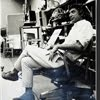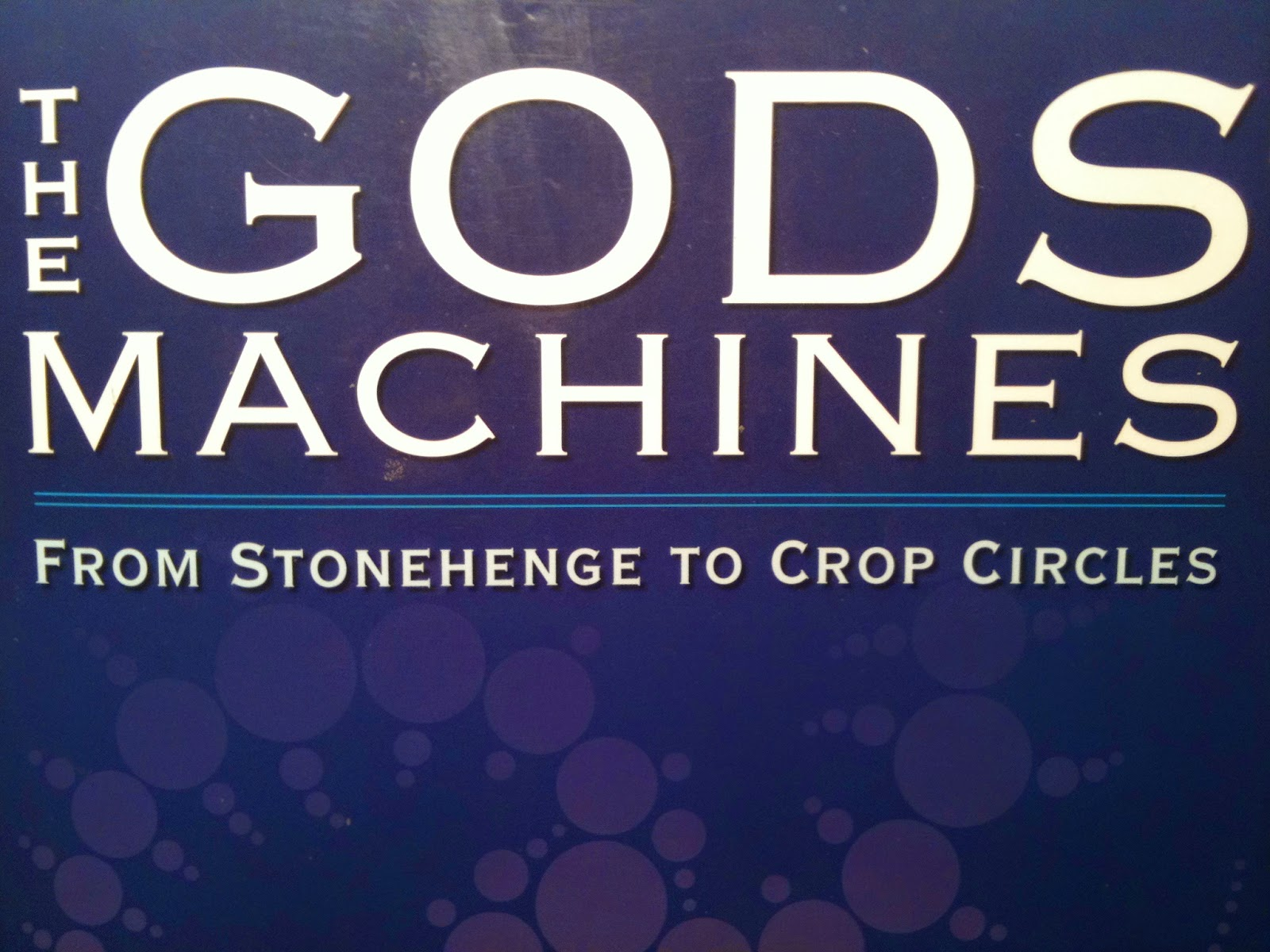## Eugene Ray SDSU 1970'sEugene Ray 1970's. photo credit: Tom Davis

## Thursday, September 25, 2014

### THE GOD'S MACHINES / radiant matrix for our future

+"ONLY THE FANTASTIC IS POSSIBLE+
>(teilhard de chardin, s.j.)<
+AT THE TRANSCENDENTAL LEVEL"+
^^^^^^^^^^^^^^^^^^^^^^^^^^^^^
>mathew higgins, new professor of design<
>at sdsu asked me if i was writing a book<
+(I AM VERY GRATEFUL+
+>FOR HIS INTEREST)+
>about the contents of this nightly blog<
^^^^^^^^^^^^^^^^^^^^^^^^^
+MY RESPONSE IS, "I SIMPLY+
+DON'T HAVE THE TIME TO+
+WRITE BLOG+BOOK TOO"+
^^^^^^^^^^^^^^^^^^^^^
>my hope is that someone will produce<
>a good book based on this blog's data<
+(PROF ROB. RAY OF SDSU+
+ARCHIVES HAS DONE AN+
+EXELLENT JOB /(conserva.)+ !
>focusing archival content w/ empathy<
^^^^^^^^^^^^^^^^^^^^^^^^^^
+(CURRENT REFERENCE)+
>a recent book on the history of rock<
>music sounds to be of high quality<
+FACTOR OF COST+
>(a problem is that it sells for \$175.<
>perhaps a condensed economy<
>version also could be printed)<
^^^^^^^^^^^^^^^^^^^^^^^

+"THE GODS MACHINES"+
>wun chok bong's monumental research<
>into the history of biotronic energy as<
+(>THOROUGH AND+
+ACCURATE BOOK)+
>used in architectural history is a fine<
>ref. to editing a book on my work<
^^^^^^^^^^^^^^^^^^^^^^>the impressive data in this work stands alone<
>in its exhaustive thoroughness & supported<
+in ARCHITECTURE)+
>by the research of experts like dr. patrick<
>flanigan, david childress & chris dunn<
^^^^^^^^^^^^^^^^^^^^^^^^^

>google provides an extensive collection of photos<
>extracted from my blog that now has over >700<
+(GRATITUDE TO ALUM /+
+>FRIEND, DAVE FOBES)+ !
>blogs published with over >55,000< viewers<
^^^^^^^^^^^^^^^^^^^^^^^^^^^
>alumni like howard spellman who i designed<
>this earth sheltered house for and is now an<
+(NO BOXES FOUND+
+in EARTH NATURE)+
>experienced builder can make a vital mark<
>using concepts that i have given freely<
^^^^^^^^^^^^^^^^^^^^^^^^^
>in about 1994 not long before i retired, a<
>handful of our students won first place<
+("SPORTSHOUSE" WINS+
+> IN SAN LUIS OBISPO)+
>in a cal poly "poly royale" compétition<
>w/ this openable "unisphere" concept<
^^^^^^^^^^^^^^^^^^^^^^^^^^^
>a light weight tensile lamella roof covers<
>an archaeology dig on the presidio hill<
+(LAMELLA FRAMING+
+FOR TENSILE ROOF)+
>of san diego in my sdsu service design<
>(one of many public service projects)<
^^^^^^^^^^^^^^^^^^^^^^^^^^^
>this research of christopher dunn supports<
+EGYPT-ET SYNERGY+
>the research of wun chok bong's books<
^^^^^^^^^^^^^^^^^^^^^^^^
>i have just acquired an encyclopedia devoted<
>to egyptian history published in england that<
+(EGYPTIAN CIVILIZATION+
+WAS VERY IMPRESSIVE)+
>is impressive w/ an in-depth overview that<
^^^^^^^^^^^^^^^^^^^^^^^^^^^^
>architectural history shall support my vision<
>of better, stronger, spherical biotronic design<
+FOR FUTURE LIVES)+
>in affordable housing for future generations<
^^^^^^^^^^^^^^^^^^^^^^^^
>this ancient solar device is one of many finds<
>around the world verifying the et presence on<
+(EXTRATERRESTRIAL+
+VISION / SUPERB AID)+
>planet earth of intelligent cosmic interface<
>(note: indian govt. mars orbiter is active)<
^^^^^^^^^^^^^^^^^^^^^^^^^^^
>"red rest" bungalow at the cove beach in lajolla<
>was affordable healthy housing that we have<
+(CALIFPRNIA BUNGALOW+
+>AFFORABLE, HEALTHY)+
>lost except for radiant architecture concepts<
^^^^^^^^^^^^^^^^^^^^^^^^^^^
>for over a quarter century in the silver ship<
>housing prototype concepts flowed from<
+(CALIFORNIA VILLA+
+PLANTATION 1982)+
>my drawing board to suggest potentials,<
>(w/ comprehension of being an agent<
^^^^^^^^^^^^^^^^^^^^^^^^^^
+>THIS NIGHTLY BLOG IS+